Physical Interpretations of Relativity Theory-IX

Sept. 3-6,2004 Imperial College, London

The Einstein-Myth and the Crisis in Modern Physics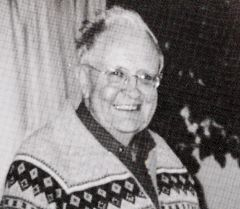F. Winterberg University of Nevada Reno, Nevada USA

Abstract

Modern physics consists of two paradigms and one myth: The theory of relativity, quantum theory and the Einstein myth. While both, the special theory of relativity and quantum mechanics, are confirmed by a very large body of experimental facts, this cannot be said about the general theory of relativity. But it is the general theory of relativity and gravitation which has created the Einstein myth through the fascination of the non-euclidean geometry adopted by Albert .Einstein from his German landsman Bernhard Riemann.

It is the failure to quantize Einstein's gravitational field theory formulated in a Riemannian curved space-time which has led to a profound crisis in modern physics, no less profound than was the crisis of physics at the beginning of the 20th century, resolved by the special theory of relativity and quantum mechanics.

To overcome the present crisis several leading theoretical physicists have entered a maze of speculations from which there appears to be no escape: The conjectured existence of higher dimensional spaces, previously reserved by the spiritists as the seat for the ghosts of the dead, not supported by a single piece of physical evidence, with all physics laboratories still three-dimensional.

When Heisenberg unsuccessfully tried to formulate a unified theory of elementary particles he only tried to change the postulates of quantum mechanics, but did not question the special theory of relativity. This example shows that the question to be asked should be: Could it be that either quantum mechanics or the theory of relativity, or perhaps both be "wrong," in the same sense "wrong" as Newton's mechanics was found to be "wrong" in the face of quantum mechanics?

In my talk I will present compelling reasons why the special theory of relativity, and by implication the general theory of relativity, cannot be the ultimate truth describing the physical universe. And the same must be said about quantum mechanics with its strange, over 10 meters experimentally verified, superluminal quantum correlations.

We only know with some certainty that physics must have its roots at the Planck energy of ~ 1019 GeV. This view is also shared by the string- and M-theorists. Even though this energy is inaccessible to particle accelerators, it is not inaccessible to our imagination, but a theory formulated at the Planck energy must be capable to reproduce all the known facts of low energy physics, like the masses of all elementary particles and the coupling constants as the finestructure constant.

In opposition to the string- and M-theorists, who got stuck in their higher dimensional speculations, a growing number of physicists are, in what is called "Analog Models of General Relativity," trying to understand gravity, not with a curved space-time, but in a more conventional way with analogs taken from condensed matter physics. Following this line of thought I have tried not only to find a condensed matter analog of general relativity, but also of quantum mechanics. This model is formulated at the Planck energy of ~ 1019 GeV, and it can at least in a qualitative way explain all the features of the standard model of elementary particle physics including gravity.

1. Introduction

It was Einstein's obsession that geometry is marble and matter is wood, and that all attempts to find the fundamental law of nature should be directed by the quest to turn wood into marble. In the context of the general theory of relativity marble means the non-Euclidean structure of space-time, while in the context of quantum mechanics matter means atoms. In contrast to Einstein, who believed that the fundamental law can be found in geometry, it was Heisenberg's belief that it should be found in quantum mechanics, that is in an atomic structure. It is the same ancient schism between Plato and Democritus, with Plato believing in geometry as the fundamental truth and Democritus believing that everything can be explained by atoms embedded in empty space.

In contradiction to Einstein there is no logical reason why everything should be reduced to marble, i.e. geometry and not to wood, i.e. atoms. While Heisenberg failed in his attempt to reduce all the laws of physics to an atomic structure with a nonlinear spinor theory, Einstein's epigones still seek to find the answer in higher dimensions of space, at the last count (F-theory) a total of 12 dimensions, 10 space and 2 time dimensions. Heisenberg failed because of unavoidable divergences, which can be seen to result from the special theory of relativity even Heisenberg did not dare to question. The adding of even more dimensions, done by the epigones, is reminiscent of the numerous attempts by the epigones of Ptolomaeus to save his system by adding an increasing number of epicycles, which led them into a maze from which there was no escape, and I predict the same fate for the epigones of Einstein.

The Aristotelean myth was a geometric universe ruled by the laws of Euclidean geometry, with the axiom of circular motions around the earth. The Einstein myth is a non-Euclidean universe with three axioms:

1. The velocity of light is constant and equal to c in all inertial references systems.
2. The principle of relativity.
3. The equivalence principle.

The Ptolemaic system was overcome by a simplifying principle placing the sun in the center, and I predict a future theory with a novel simplifying principle will derive Einstein's axioms from a more fundamental level.

2. Einstein's Program - The March of the Geometries or Making Marble out of Wood

The Ptolemaic system was cast in the concrete of circular motions, permitting us to add an arbitrary number epicycles. In a similar way Einstein's universe is cast in the concrete of geometry, permitting us to add an arbitrary number of (higher) dimensions.

In the gravitational field equations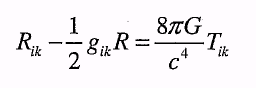(1)

geometry is expressed by the curvature tensor on the left hand side, and matter by the energy momentum tensor on the right hand side, with Newton's constant G a measure of the strength with which matter curves space-time. The trajectory of a test particle in the gravitational field obtained as a solution of (1) is a geodesic given by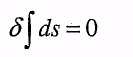(2)

where ds is the four dimensional line element of the Riemannian non-Euclidean metric. Compare this with quantum theory where the force is explained by the exchange of "virtual" particles, borrowing energy from the vacuum with Heisenberg's uncertainty principle. Einstein's program is to bring the r.h.s. of (1) onto the l.h.s. by also giving the r.h.s. a geometric meaning. For this to be done, it has first to be recognized that the r.h.s. contains two very different parts, one from bosonic and the other from fermionic fields, making up the so-called standard model. The bosonic part consists of the electromagnetic field, the strong and weak interaction fields, and the fermionic part of the quark and lepton fields. The bosonic fields, in particular the electromagnetic field, have the character of force fields, whereas the fermionic fields are representative of a particle described by the Dirac equation. At first, and that is what Einstein tried to do is to bring the bosonic part to the l.h.s. of eq. (1), describing the trajectory of a charged particle in an electromagnetic field for example, by a geodesic equation in a curved space as it is done for an uncharged particle in a gravitational field. To do this is relatively easy. It is more difficult to bring the fermionic fields to the l.h.s. of (1) as well. Ignoring quantum mechanics three steps were taken:

1. By adding a fifth space dimension (Kaluza-Klein), Maxwell's equation could be incorporated into the spacetime curvature tensor on the l.h.s., whereby the trajectory of an electrically charged particle could be described as a geodesic in 5-dimensional spacetime.
2. By further increasing the number of dimensions, Yang-Mills theories, describing the weak and strong nuclear interactions could likewise be brought to the l.h.s. of (1).

3. By doubling the four spacetime dimensions of the curved Riemannian space, where the coordinates are ordinary commuting numbers like 3x4 = 4x3, resp. ab = ba, with four space-time dimensions where the coordinates are anticommuting Grassmann numbers, ab = —ba (numbers which can be expressed by matrices), both bosonic (corresponding to ordinary numbers), and fermionic (corresponding to Grassmann numbers) fields could be given a geometric meaning.

The third, all encompassing step led to the theory of supergravity. Symbolically one may write:

Riemann + Einstein = Gravity

Riemann + Grassmann+Einstein = Supergravity.

This completes Einstein's program for a unified classical field theory for all fields in physics, or the conversion of all "wood" into "marble." But because it leaves out quantum mechanics it cannot be complete, and it is here where supergravity fails. Therefore, supergravity was not the end of physics as media-celebrity physicist Steven Hawking once believed it was.

Finally, an irony: Grossmann brought the work of Einstein's landsman Riemann to the attention of Einstein, which gave Einstein the decisive clue to solve the problem of gravity. If someone else had brought the work of Einstein's other landsman Grassmann to Einstein's attention, Einstein could have discovered the theory of supergravity a long time ago, and fulfilled his lifelong dream for a unified field theory.

3. The Problem of the Infinities

"Let me say something that people who worry about mathematical proofs and inconsistencies seem not to know. There is no way of showing mathematically that a physical conclusion is wrong or inconsistent. All that can be shown is that the mathematical assumptions are wrong. If we find that certain mathematical assumptions lead to a logically inconsistent description of Nature, we change the assumptions, not Nature."

Richard P. Feynman, Feynman Lectures on Gravitation, Addison-Wesley Publishing Co. New York, 1965 p. 183.

The reason why quantum theory prevents supergravity to be the final theory is because its quantization leads to infinite results. It is often incorrectly claimed that the problem of infinities already occurs in classical electrodynamics in computing the energy, and hence mass, of a point charge. This claim is quite incorrect, because Maxwell's equations have no point charge solutions.

Rather, the following is true:

1. A mechanism with a finite number of degrees of freedom, for example an atom, if quantized has a discrete set of energy levels. And a field, for example Maxwell's electromagnetic field, can be interpreted as a mechanism with an infinite number of degrees of freedom, which if quantized leads to photons as the quanta of this field. Similarly, Dirac's equation can be viewed as a classical field equation, which if quantized leads to electrons as the quanta of this field.
2. The theory of relativity requires that the particles of the quantized field must be outlike,
because the time sequence of cause and effect can otherwise be changed by a Lorentz transformation.

An extended particle, to be called elementary, would have to be rigid, where a signal passing through the particle is infinite, i.e. superluminal, which according to the theory of relativity would violate causality in the sense that an effect must always follow the cause in any reference system. Therefore, extended particles are always composed of some smaller particles, or what is the same, held together by fields which if quantized would have pointlike particles as the quanta of this field. A good example for an extended particle is a proton which is composed of three pointlike quarks held together by the field of gluons. The infinities then simply follow from the application of Heisenberg's uncertainty principle, which says that for a vanishing length, i.e. point, the energy fluctuation diverges inversely proportional to the length.

In the two most important field theories of matter:

1. Quantum electrodynamics, describing the force between electrons by the electromagnetic force, and
2. Quantum chromodynamics, describing the force between quarks by the chromodynamic force, the electrons, resp. quarks, are surrounded by virtual electron-positron, resp. quark-antiquark parrs, screening the electric charge of an electron, resp. anti-screening the chromodynamic charge of a quark.

In both cases this makes the infinite selfenergy diverge only logarithmically. Primarily because of this weak divergence, one can, with the so-called renormalization procedure, isolate the divergence in a relativistically invariant way.

It roughly works as follows: The experimentally known particle mass (resp. energy) is assumed to be the difference of two infinite quantities, the infinite unrenormalized mass of the particle, and the infinite energy stored in the field surrounding the porntlike particle. In these renormalizable theories, virtual particle pairs borrow their energy for a short time out of the vacuum with the help of Heisenberg's uncertainty principle. With no comparable virtual pair production in a theory of quantum gravity, Einstein's general theory of relativity and gravitation, but also supergravity cannot be renonnalized and quantized. According to Feynman this simply means that the mathematical assumptions are wrong. These assumptions are here Einstein's gravitational field equations and quantum mechanics. Therefore, either one of them or both cannot be completely correct. Here is where string theory claims to provide an answer. Qualitatively one can say this: In classical electrodynamics the selfenergy of an electrically charged sphere is inversely proportional to the diameter of the sphere, and the selfenergy of a string is proportional to the logarithm of its diameter. With the selfenergy of a charged sphere reduced by electron positron pair production to a logarithmic dependence, it seems plausible that for a charged string the selfenergy is finite. However, this is really not true because the divergent selfenergy is there compensated by the infinite stress in the zero diameter string.

4. Unphysical Properties of Einstein's Marble

In the special theory of relativity any object is described by a world line in a four dimensional space. It is the Minkowski spacetime, encompassing the past, present and future. With the velocity of light the highest possible velocity, a world line must be positioned inside the light cone, and there can be no closed world line because it would have to pass through forbidden regions outside the light cone, requiring superluminal velocities. But as it was first shown by Goedel, what is not possible in the flat spacetime of special relativity, is possible in the curved spacetime of general relativity. Solutions with closed world lines occur in a rotating universe, but also in the vicinity of rotating black holes. Solutions with closed world lines, make possible travel back in time, obviously not possible hi physical reality. Other solutions of general relativity discovered by Newman, Unti and Tamburino, are multivalued, which too have to be excluded from physical reality.

As beautiful as Einstein's theory is (as was the Ptolomaic wheels in wheels theory of the planetary system), it cannot be a completely correct description of physical reality. As a model it must not be totally wrong, because it can quite well describe astronomical anomalies, like the perihelion motion of Mercury, which Newton's theory was unable to explain.

5. Einstein - Parmenides and the Ontological Proof for the Non-Existence of God

The special theory of relativity understood by Einstein as a four-dimensional space-time continuum implies a kind of superdeterrninisrn with the future completely determined down to the smallest detail. This was the reason why Einstein believed time is an illusion and why Karl Popper told Einstein "You are Parmenides," the Greek philosopher (515-445) who believed that being is not becoming and time (becoming) an illusion. With everything exactly predetermined there can be no free will, not even a hypothetical God, and a God without free will is an ontological impossibility.

One therefore can say: If Einstein is right, then there can be no God. The opposite though, is not true; true rather is if God exists then Einstein must be wrong.

6. The Two Faces of Quantum Mechanics

A deeper insight is gained if one realizes that quantum mechanics has two faces:

1. The deterministic evolution of the wave function describing a particle moving with subluminal
velocities inside the light cone.
2. The deterministic collapse of the wave function going with superluminal velocity outside the light cone.

Whereas for the deterministic evolution of the wave function there exists a well developed theory (Schrodinger and Dirac equation) no such theory exists for the superluminal wave function collapse.

Because the superluminal collapse occurs in the course of making a measurement, this is wrongly called the measurement problem, with the claim that the Copenhagen interpretation of quantum mechanics provides the missing theory. This is also the position taken by the string theorists. Taking instead the position that the wave function is an object of physical reality, not just an expression of our limited knowledge of reality as the Copenhagen interpretation claims, the occurrence of the superluminal wave function collapse (with certainty observed over -10 meters in the experiment by Aspect), is in gross violation of Einstein's light velocity postulate, and it is only through the stochastic nature of the wave function collapse that a peaceful coexistence of quantum mechanics with the special theory of relativity seems possible.

A deterministic theory of this collapse, required if the wave function is real, would destroy this coexistence and with it special theory of relativity and the Minkowski spacetime. And it would also bring down string theory and its latest successor, the M-theory.

But if the wave function is real and its collapse going with superluminal velocity, the straight]acket of the Minkowski spacetime is broken, reestablishing the possibility of a free will and a God, impossible in Minkowski spacetime.

7. On Mach's Principle and Minkowski Space-time

Mach's principle is the conjecture that inertia has its cause in the accelerated motion relative to all masses in the universe. According to this conjecture the Coriolis and centrifugal forces observed on a rotating platform would be the same if all the masses of the universe are brought into a rotational motion around the platform. Support for this conjecture seemed to be provided when Thirring (Physik. Z. 19, 33 (1918)) showed that Einstein's gravitational field equations, applied to the space inside a hollow sphere of mass M and radius R set into rotation around its axis with the angular velocity a, predict in the center of the sphere a force (per unit mass)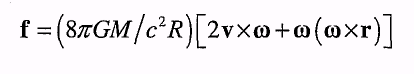(3)

Setting R equal the world radius R = 8ftGM/c2, with M the mass of the universe, this force becomes the same as the Coriolis and centrifugal force observed on a rotating platform. But it would take the time R/c after the cosmic sphere is set into rotation, that is billions of years, before the force f is felt in the center of the sphere of radius R . Thirring' s solution therefore is not a derivation of Mach's principle from general relativity. In fact, inertial forces occur even in Minkowski spacetime void of any matter, making Newton's absolute space true as ever.

Overlooked is the zero point vacuum energy. With inertia "present," and not transmitted with a long tune delay as in Thirring's solution, it can only be the vacuum energy that is responsible for the phenomenon of inertia. As quantum mechanics tells us this vacuum energy has a divergent of frequency spectrum. If cut off at the Planck length it gives the vacuum a mass density of ~ 1095 g/cm3. It is this huge mass density which can explain why inertia is highly isotropic. At

Mach's time the vacuum energy was not known. With the vacuum energy, complete kinematic equivalence would then be achieved if not only all the masses of the universe are brought into a rotational motion around a stationary platform, but also the vacuum energy.

The of frequency dependence of the zero point vacuum energy is the only one which is Lorentz invariant. This suggests that it must be the vacuum energy which "erects" the Minkowki spacetinie. But because the vacuum energy has to be cut off at the Planck length, Lorentz invariance is broken, establishing a privileged reference system at rest with the vacuum energy. As Selleri has shown the slightest violation of Lorentz invariance, no matter how small, will ultimately bring down the whole edifice of special relativity and by implication of general relativity, as it was feared by Einstein shortly before his death in a letter to his friend M. Besso.

The conclusion that the zero point vacuum energy must be the reason why Minkowski space-time as an approximate description of physical reality, is supported by the Scharnhorst effect. This effect predicts a small increase in the velocity of light by a reduction of the zero point vacuum energy as it occurs in the Casimir effect.

Now we can understand why Heisenberg failed in his attempt to reduce all marble to wood. In Einstein's program the Minkowski space-time is the most elementary form of marble, with general relativity given the marble of space-time only a different shape. If Heisenberg's goal was to reduce all marble to wood, he should have done the same with space-time, or what is the same reduce space-time to atoms. "Atoms," of course, in the finitistic sense, as opposed to continuous.

8. Non-Euclidean is Marble - Non-Archimedean is Wood

"I consider it quite possible that physics cannot be based on the field concept, i.e., on continuous structures. In that case, nothing remains of my entire castle in the air, gravitational theory included, [and of] the rest of modern physics." A. Einstein (1954) in a letter to his friend M. Besso.

In his marble versus wood analogy Einstein understood marble as a metaphor for non-Euclidean geometry, but it seems that he never asked himself the question what would be the metaphor of wood. I make the claim that it is a metaphor for what mathematicians call non-Archimedian geometry.

Archimedes believed he could determine the value of K through a limiting process, by drawing a sequence of polygons inside a circle with an ever increasing number of sides. This "exhaustion" method though must fail if there is a smallest length. It was Planck who in a 1899 paper had shown that the fundamental constants of physics, h, G and c, give us such a small length, the Planck length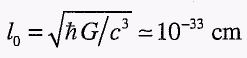,

in addition to a fundamental mass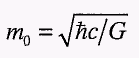and fundamental time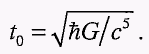These three quantities are sufficient for the architecture of a non-Archimedean geometry for a finitistic formulation of physics. The square root in the expression for m0 gives us only the freedom to have two possible signs for m0, but nothing more.

In such a finitistic formulation one can, in an arbitrary number of space dimensions, replace differentiation operators by finite difference operators.

9. Planck Mass Plasma

With Planck's finite size elements of space, time and mass, the most simple configuration one can think of is what one may call a Planck mass plasma. It makes the following three assumptions:

1. Space is densely filled with an equal number of positive and negative Planck mass particles, with each Planck length volume element occupied by one Planck mass particle.
2. The Planck mass particles interact over a Planck length with the Planck force c4/G, with particles of equal sign repelling, and those of opposite sign attracting each other.
3. The interaction obeys the laws of Newtonian mechanics, except for lex tertia, which under the assumed force law is violated during the collision between a positive and a negative Planck mass particle.

The violation of the lex tertia means that during the mutually attractive collision between a positive and a negative Planck mass particle, the momentum, not the energy, fluctuates. This establishes Heisenberg's uncertainty principle at the most fundamental level, explaining why quantum mechanics can be derived from the Planck mass plasma.

In addition to quantum mechanics the Planck mass plasma leads to Lorentz invariance as a dynamic symmetry, with a spectrum of quasiparticles greatly resembling the particles of the standard model. It also gives a novel perspective on Einstein's quest to unify gravity with electromagnetism, with gravity and electromagnetic waves interpreted as the symmetric and antisymmetric vortex lattice wave modes of the superfluid Planck mass plasma. And because of the fluid dynamic analogy, it also leads the principle of equivalence.

Furthermore, Dirac spinors can be explained as excitonic particles made up from the positive and negative mass component of the Planck mass plasma, with the compensating effect of the negative masses explaining the smallness of the typical fermion mass hi terms of the Planck mass. Finally, the Planck mass plasma may conceivably be able to explain the superluminal wave function collapse as a gravitational collapse enhanced by the hidden presence of negative masses.

10. On Quantum Gravity

One of the major outstanding problems of modern physics is quantum gravity. Because it leads to nonrenormalizable infinities, Einstein's gravitational field equations cannot be quantized. This is possible with string (M) theory, but the price to be paid is high: It is the need to assume the existence of the higher dimensions, in particular 10 spacetime dimensions. But with physical reality taking place in 4 spacetime dimensions, the superfluous 6 dimensions have to be compactified, with physics suggesting that they have to be compactified down to the Planck length of ~ 1CT33 cm. Since this can be done in a very large number of different ways, each leading to a different universe, the uniqueness of the theory is lost.

In the Planck mass plasma, where gravity is associated with a transverse vortex lattice wave, which for small amplitudes has the same property as Einstein's gravitational waves in the weak field limit of general relativity, the situation is quite different. In this theory, special relativity, and by implication general relativity, is a dynamic symmetry as in the pre-Einstein theory of relativity by Lorentz and Poincare. It assumed the existence of an aether, taken up by the Planck mass plasma. With the Planck mass plasma made up of discrete elements for which the laws of Newtonian mechanics apply, there can be no infinities, as in the many body problem of nonrelativistic quantum mechanics. It appears to the author that such a finitistic description of physical reality is more plausible than the assumption of higher dimensions.

A comparison of the string- and Planck mass plasma model is also instructive. In the Planck mass plasma, the zero diameter strings in 9 space and one time dimension, are replaced by vortices in 3 space and one time dimension, with a diameter of the vortex core equal one to Planck length. And the closed strings with a ring radius equal to the Planck length are replaced by vortex rings, with a ring radius about thousand times larger than the Planck length. In both models, gravitational waves are described by the same kind of elliptic deformations of the closed strings resp. vortex rings.

For the details of the Planck Mass Plasma model I may direct the interested reader to my paper "Planck Mass Plasma Vacuum Conjecture", Z. Naturforsch. 58a, 231-267 (2003), and other references, there quoted.